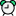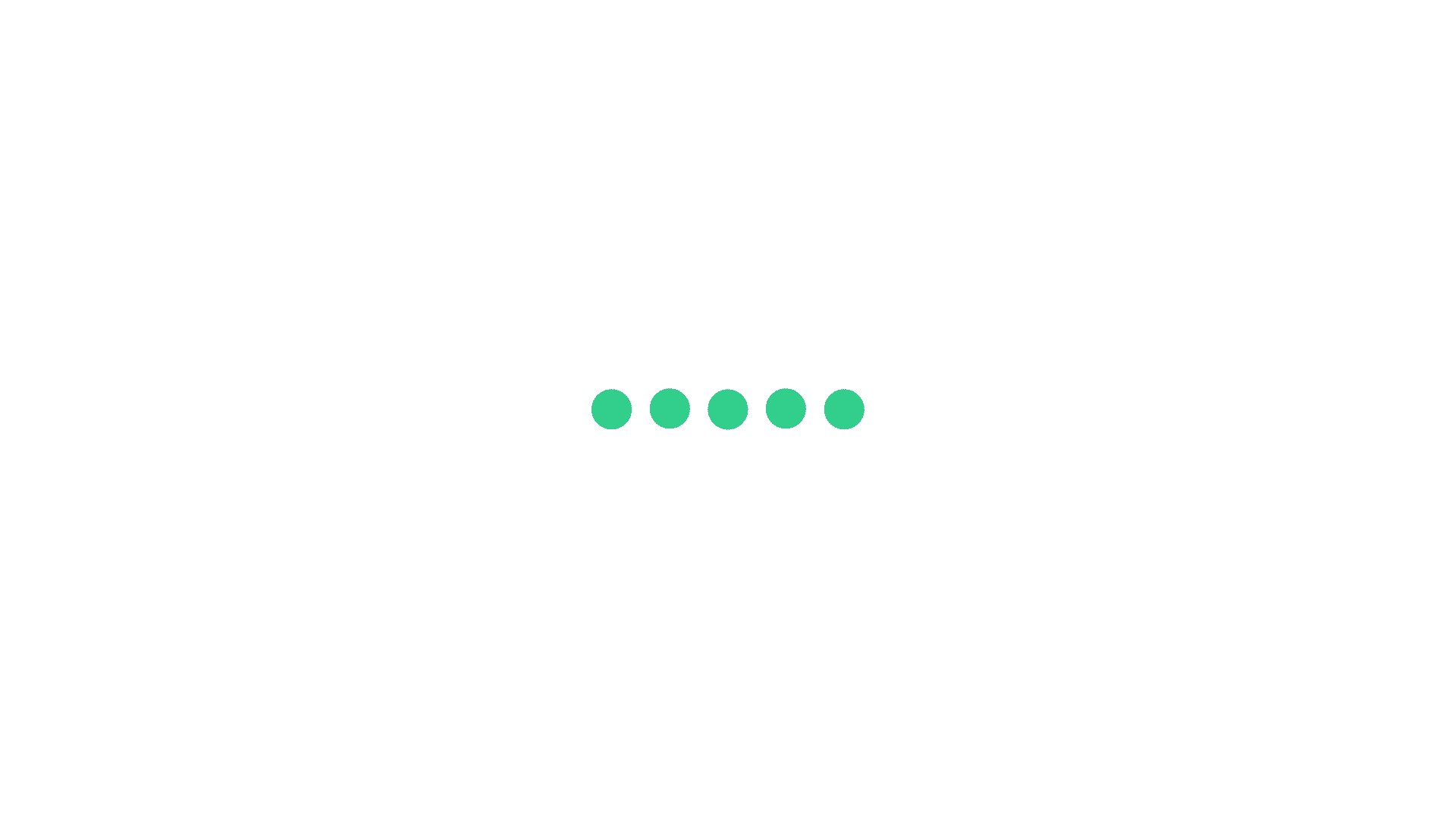Time: 00:00:00

Question #1

The LCM of three different numbers is 2048. Which one of the following can never be there HCF?A
8
8
B
124
124
C
32
32
D
256
256
Once you attempt the question then PrepInsta explanation will be displayed.

StartTime: 00:00:00

Question #2

The least perfect square, which is divisible by each of 20, 35 and 65 isA
3314434
3314434
B
3341200
3341200
C
3312400
3312400
D
4331444
4331444
Once you attempt the question then PrepInsta explanation will be displayed.

StartTime: 00:00:00

Question #3

Ram got a question in his maths test that,If LCM of two number is 369, HCF of two numbers is 27and one number is 9, then find otherA
390
390
B
1212
1212
C
1107
1107
D
1502
1502
Once you attempt the question then PrepInsta explanation will be displayed.

StartTime: 00:00:00

Question #4

Find the greatest number which on dividing 1654 and 2153, leaves a remainder of 24 and 23 respectively?A
15
15
B
12
12
C
10
10
D
13
13
Once you attempt the question then PrepInsta explanation will be displayed.

StartTime: 00:00:00

Question #5

Which greatest possible length can be used to measure exactly 15 meter 75 cm, 11 meter 25 cm and 7 meter 65 cm ?

Which greatest possible length can be used to measure exactly 20 meter 15 cm, 21 meter 55 cm and 12 meter 65 cm ?A
8
8
B
5
5
C
4
4
D
2
2
Once you attempt the question then PrepInsta explanation will be displayed.

StartTime: 00:00:00

Question #6

Five clothes dry in 3,6,9,12 and 15 hours respectively, how many times will these clothes will be dried in 360 hoursA
5
5
B
4
4
C
3
3
D
2
2
Once you attempt the question then PrepInsta explanation will be displayed.

StartTime: 00:00:00

Question #7

There are 3 numbers, these are co-prime to each other are such that the product of the first two is 399 and that of the last two is 525. What will be the sum of three numbers :A
75
75
B
81
81
C
65
65
D
89
89
Once you attempt the question then PrepInsta explanation will be displayed.

StartTime: 00:00:00

Question #8

If the sum of two numbers is 11 and the H.C.F. and L.C.M. of these numbers are 1 and 30 respectively, then the sum of the reciprocals of the numbers is equal to:A
55/60
55/60
B
60/55
60/55
C
11/30
11/30
D
10/11
10/11
Once you attempt the question then PrepInsta explanation will be displayed.

x+y=55 xy=600 1/x+1/y=11/120

StartTime: 00:00:00

Question #9

What is the smallest number that leaves a remainder of 2 when divided by 3, 4, 5, and 6?A
62
62
B
46
46
C
32
32
D
25
25
Once you attempt the question then PrepInsta explanation will be displayed.

StartTime: 00:00:00

Question #10

Find the smallest 4 digit number which is divisible by 18, 24, 32?A
1152
1152
B
234
234
C
123
123
D
145
145
Once you attempt the question then PrepInsta explanation will be displayed.

Start

["0","40","60","80","100"]
["Need more practice! \r\n \r\n \r\n","Keep trying! \r\n \r\n \r\n","Not bad! \r\n \r\n \r\n","Good work! \r\n \r\n \r\n","Perfect! \r\n \r\n \r\n"]Join Wipro Online Classes#### Your Quiz 1 percentile Percentile (0%)

-

-